Friday , October 7 2022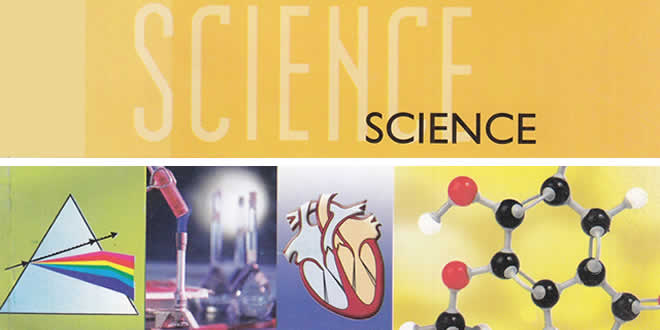# Light Reflection and Refraction MCQs: 10 Science Ch 10

Light Reflection and Refraction MCQs: CBSE Class 10 Science Chapter 10 Light Reflection and Refraction Multiple Choice Questions with Answers. MCQ Questions for Class 10 Science with Answers was prepared based on latest exam pattern. Students can solve NCERT Class 10 Science Light Reflection and Refraction Multiple Choice Questions with Answers to know their preparation level.

 Class: 10th Class Subject: Science Chapter: Chapter 10: Light Reflection and Refraction Quiz: – Questions MCQs: 95 Questions

## Light Reflection and Refraction MCQs

### Class 10 Science Light Reflection and Refraction MCQs with Answers & Explanation:

#### 1. An object is placed at a distance of 0.25 m in front of a plane mirror. The distance between the object and image will be

(a) 0.25 m
(b) 1.0 m
(c) 0.5 m
(d) 0.125 m

Explanation: (c) Distance between object and image = 0.25 + 0.25 = 0.5 m

#### 2. The angle of incidence for a ray of light having zero reflection angle is

(a) 0
(b) 30°
(c) 45°
(d) 90°

Explanation: (a) For reflecting surface ∠i = ∠r

#### 3. For a real object, which of the following can produce a real image?

(a) Plane mirror
(b) Concave mirror
(c) Concave lens
(d) Convex mirror

Light Reflection and Refraction MCQs – Answer: b
Explanation: (b) Only concave mirror can produces a real image for the any position of object between its focus and infinity.

#### 4. Which of the following mirror is used by a dentist to examine a small cavity?

(a) Convex mirror
(b) Plane mirror
(c) Concave mirror
(d) Combination of convex and concave mirror

Explanation: (c) Concave mirror forms erect and enlarged image when held close to the cavity.

#### 5. An object at a distance of 30 cm from a concave mirror gets its image at the same point. The focal length of the mirror is

(a) – 30 cm
(b) 30 cm
(c) – 15 cm
(d) +15 cm

Explanation: (c) When object is placed at 2F, the image formed by concave mirror is also at 2F.
So 2F = -30 or F = -15 cm.

#### 6. An object at a distance of +15 cm is slowly moved towards the pole of a convex mirror. The image will get

(a) shortened and real
(b) enlarged and real
(c) enlarge and virtual
(d) diminished and virtual

Explanation: (d) Convex mirror always formed virtual and diminished image.

#### 7. A concave mirror of radius 30 cm is placed in water. It’s focal length in air and water differ by

(a) 15
(b) 20
(c) 30
(d) 0

Explanation: (d) The focal length of spherical mirror does not depends on the surrounding medium.

#### 8. A concave mirror of focal length 20 cm forms an image having twice the size of object. For the virtual position of object, the position of object will be at

(a) 25 cm
(b) 40 cm
(c) 10 cm
(d) At infinity

Explanation: (c) For virtual image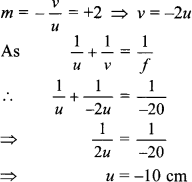#### 9. The image formed by concave mirror is real, inverted and of the same size as that of the object. The position of object should be

(a) at the focus
(b) at the centre of curvature
(c) between focus and centre of curvature
(d) beyond centre of curvature

Explanation: (c) When object lies at C of a concave mirror, image is also formed at ‘C’ and having same size real and inverted.

#### 10. The nature of the image formed by concave mirror when the object is placed between the focus (F) and centre of curvature (C) of the mirror observed by us is

(a) real, inverted and diminished
(b) virtual, erect and smaller in size
(c) real, inverted and enlarged
(d) virtual, upright and enlarged

Explanation: When object lies between C and F, the real, inverted and enlarged image is formed beyond C.

### Class 10 Science Light Reflection and Refraction MCQs with Answers & Explanation:

#### 11. The nature of image formed by a convex mirror when the object distance from the mirror is less than the distance between pole and focal point (F) of the mirror would be

(a) real, inverted and diminished in size
(b) real, inverted and enlarged in size
(c) virtual, upright and diminished in size
(d) virtual, upright and enlarged in size

Explanation: Convex mirror always forms a virtual, erect diminished image irrespective of the position of object in front of it.

#### 12. If a man’s face is 25 cm in front of concave shaving mirror producing erect image 1.5 times the size of face, focal length of the mirror would be

(a) 75 cm
(b) 25 cm
(c) 15 cm
(d) 60 cm

Explanation: In concave shaving mirror, virtual erect and large size image, behind the mirror is obtained, using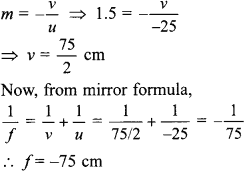Hence, focal length of concave mirror is 75 cm.

#### 13. As light travels from a rarer to a denser medium it will have

(a) increased velocity
(b) decreased velocity
(c) decreased wavelength
(d) both (b) and (c)

Explanation: When light ray travel from rarer to denser medium, its velocity and wavelength both decrease as v = vλ.

#### 14. The angle of incidence i and refraction r are equal in a transparent slab when the value of i is

(a) 0°
(b) 45°
(c) 90°
(d) depend on the material of the slab

Light Reflection and Refraction MCQs – Answer: a
Explanation: When the incident ray falls normally on the glass slab, it will refracted without deviation, i.e. along the normal in the glass slab. So, ∠i = ∠r = 0

#### 15. The refractive index of transparent medium is greater than one because

(a) Speed of light in vacuum < speed of light in transparent medium
(b) Speed of light in vacuum > speed of light in transparent medium
(c) Speed flight in vacuum = speed of light in transparent medium
(d) Frequency of light wave changes when it moves from rarer to denser medium

Explanation: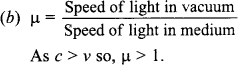#### 16. The refractive index of water is 1.33. The speed of light in water will be

(a) 1.33 × 108 m/s
(b) 3 × 108 m/s
(c) 2.26 × 108 m/s
(d) 2.66 × 108 m/s

Explanation: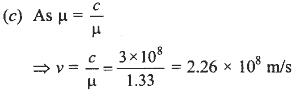#### 17. You are given three media A, B and C of refractive index 1.33, 1.65 and 1.46. The medium in which the light will travel fastest is

(a) A
(b) B
(c) C
(d) equal in all three media

Explanation: So, the light will travel faster in a medium having lower refractive index.

#### 18. Light from the Sun falling on a convex lens will converge at a point called

(a) centre of curvature
(b) focus
(c) radius of curvature
(d) optical centre

Explanation: (b) The parallel ray coming from the sun, after refraction through the convex lens converge at its focus.

#### 19. Large number of thin stripes of black paint are made on the surface of a convex lens of focal length 20 cm to catch the image of a white horse. The image will be

(a) a zebra of black stripes
(b) a horse of black stripes
(c) a horse of less brightness
(d) a zebra of less brightness

Explanation: (c) Complete image of the white horse is formed but of less intensity, the light falling on the curved portion will not react at the image position.

#### 20. A divergent lens will produce

(a) always real image
(b) always virtual image
(c) both real and virtual image
(d) none of these

Explanation: (b) Diverging (concave) lens will always forms a virtual, erect and diminished image.

## Light Reflection and Refraction MCQs

#### 21. When object moves closer to convex lens, the image formed by it shift

(a) away from the lens
(b) towards the lens
(c) first towards and then away from the lens
(d) first away and then towards the lens

Explanation: (a) As object moves from infinity towards optical centre of the convex lens image is shifted away from its focal point and towards infinity.

#### 22. When object moves closer to a concave lens the image by it shift

(a) away from the lens on the same side of object
(b) toward the lens
(c) away from the lens on the other side of lens
(d) first towards and then away from the lens

Explanation: (b) When object infinity, image is formed at the focus of concave lens of object is moved towards lens, the image is also moved from its focus towards lens and its size increase.

#### 23. A magnified real image is formed by a convex lens when the object is at

(a) F
(b) between F and 2F
(c) 2F
(d) only (a) and (b) both

Light Reflection and Refraction MCQs – Answer: d
Explanation: (d) When object is at 2F, real inverted and same size image is formed by convex lens. So, according to question option (a) and (b) both are correct.

#### 24. The distance between the optical centre and point of convergence is called focal length in which of the following cases?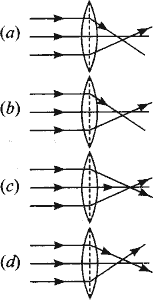Explanation: (c) Parallel rays after refracting meet at the focus of a convex lens.

#### 25. A 10 mm long awl pin is placed vertically in front of a concave mirror. A 5 mm long image of the awl pin is formed at 30 cm in front of the mirror. The focal length of this mirror is [NCERT Exemplar Problems]

(a) – 30 cm
(b) – 20 cm
(c) – 40 cm
(d) – 60 cm

Explanation: (b) Given h0 = +10 mm = + 0.1 cm
h2 = – 5 mm = -0.5 cm
for real image, v = – 30 cm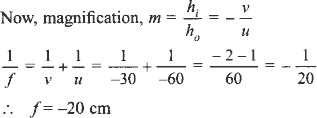#### 26. Figure shows a ray of light as it travels from medium A to medium B. Refractive index of the medium B relative to medium A is [NCERT Exemplar Problems]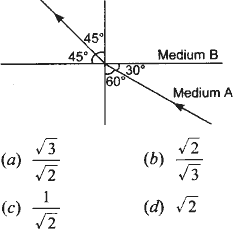Explanation: (a) Here, ∠i = 60°, ∠r = 45°
Using Snell’s law of refraction, refractive index of medium B with respect to medium A.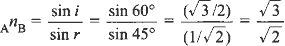#### 27. A light ray enters from medium A to medium B as shown in figure. The refractive index of medium B relative to A will be [NCERT Exemplar Problems]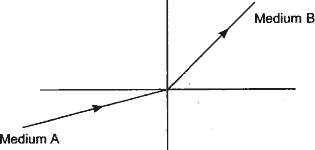(a) greater than unity
(b) less than unity
(c) equal to unity
(d) zero

Explanation: (b) In medium B, light ray bends away from the normal. It indicates that medium B is optically rarer than medium A. Hence speed of light in medium B is more than that in medium A.
Now, refractive index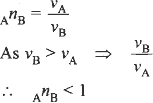#### 28. Beams of light are incident through the holes A and B and emerge out of box through the holes C and D respectively as shown in the figure. Which of the following could be inside the box? [NCERT Exemplar Problems]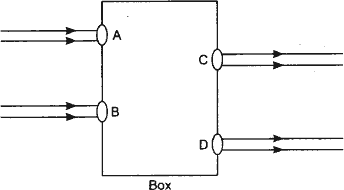(a) A rectangular glass slab
(b) A convex lens
(c) A concave lens
(d) A prism

Explanation: (a) Figure shows that emergent ray are parallel to the incident ray and shifted side ward slightly. This can be done by the rectangular glass slab only.

#### 29. A beam of light is incident through the holes on side A and emerges out of the hole on the other face of the box as shown in the figure. Which of the following could be inside the box? [NCERT Exemplar Problems]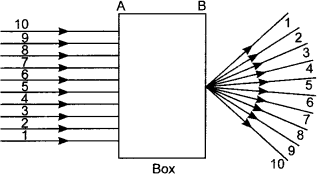(a) Concave lens
(b) Rectangular glass slab
(c) Prism
(d) Convex lens

Explanation: (d) From figure, it is clear that parallel rays converge at a point and emerges from face B. So convex lens would be possible inside the box.

#### 30. Which of the following statements is / are true? [NCERT Exemplar Problems]

(a) A convex lens has 4 dioptre power having a focal length 0.25 m
(b) A convex lens has -4 dioptre power having a focal length 0.25 m
(c) A concave lens has 4 dioptre power having a focal length 0.25 m
(d) A concave lens has – 4 dioptre having a focal 0.25 m

Explanation: (a) Positive sign with power and focal length indicates that the given lens is convex.
Also f = $$\frac{1}{P}=\frac{1}{4}$$ = 0.25m

### Class 10 Science Light Reflection and Refraction MCQs with Answers & Explanation:

#### 31. Magnification produced by a rear view mirror fitted in vehicles [NCERT Exemplar Problems]

(a) is less than one
(b) is more than one
(c) is equal to one
(d) can be more than or less than one depending upon the position of the object in front of it.

Explanation: (a) Convex mirror is used as rear view mirror and alway forms virtual, erect and diminished inage. So magnification produced by a rear view mirror is less than one.

#### 32. A full length image of a distant tall building can definitely be seen by using [NCERT Exemplar Problems]

(a) a concave mirror
(b) a convex mirror
(c) a plane mirror
(d) both concave as well as plane mirror

Explanation: (b) Convex mirror has a wide field of view as it forms virtual, erect and diminished image. Hence, fall length of image of distance tall building can definitely be seen by using it.

#### 33. In torches, search lights and headlights of vehicles the bulb is placed [NCERT Exemplar Problems]

(a) between the pole and the focus of the reflector
(b) very near to the focus of the reflector
(c) between the focus and centre of curvature of the reflector
(d) at the centre of curvature of the reflector

Explanation: (b) When source of light is placed very near to the focus of the reflector which are concave in shape, the reflected light becomes parallel to the principal axis.

#### 34. The laws of reflection hold good for [NCERT Exemplar Problems]

(a) plane mirror only
(b) concave mirror only
(c) convex mirror only
(d) all mirrors irrespective of their shape

Explanation: (d) The laws of reflection hold good for light reflected from any smooth surface irrespective of their shapes.

#### 35. The path of a ray of light coming from air passing through a rectangular glass slab traced by four students are shown as A, B, C and D in figure. Which one of them is correct? [NCERT Exemplar Problems]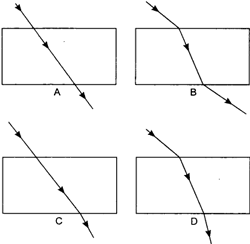(a) A
(b) B
(c) C
(d) D

Explanation: (b) When a light ray is incident oblikely on one face of rectangular glass slab, the emergent ray will be parallel to the incident ray and shifted sideward slightly.

#### 36. In which of the following, the image of an object placed at infinity will be highly diminished and point sized? [NCERT Exemplar Problems]

(a) Concave mirror only
(b) Convex mirror only
(c) Convex lens only
(d) Concave mirror, convex mirror, concave lens and convex lens.

Light Reflection and Refraction MCQs – Answer: d
Explanation: (d) The incident ray coming from the object placed at infinity will be parallel to the principal axis. When the parallel beam of light incident on a mirror or lens, irrespective of their nature, after reflection / refraction, will pass or appear to pass through their principal focus. Hence highly diminished and point size image will be formed at their focus.

#### 37. When light falls on a smooth polished surface, most of it

(a) is reflected in the same direction
(b) is reflected in different directions
(c) is scattered
(d) is refracted into the second medium

#### 38. Image formed by reflection from a plane mirror is

(a) real and inverted
(b) virtual and erect
(c) real and erect
(d) virtual and inverted

#### 39. If an incident ray passes through the focus, the reflected ray will

(a) pass through the pole
(b) be parallel to the principal axis
(c) retrace its path
(d) pass through the centre of curvature

Explanation:

#### 40. Magnifying power of a concave lens is

(a) always > 1
(b) always < 1
(c) always = 1
(d) can have any value

## Light Reflection and Refraction MCQs

#### 41. The image formed by a convex lens can be

(a) virtual and magnified
(b) virtual and diminished
(c) virtual and of same size
(d) virtual image is not formed

#### 42. A point object is placed at a distance of 20 cm from a convex mirror of focal length 20 cm. The image will form at:

(a) at infinity
(b) at focus
(c) at the pole
(d) behind the mirror

Explanation:

#### 43. Focal length of a concave mirror is

(a) negative
(b) positive
(c) depends on the position of object
(d) depends on the position of image

(a) +50 cm
(b) -100 cm
(c) -50 cm
(d) +100 cm

#### 45. A spherical mirror and a spherical lens each have a focal length of -10 cm. The mirror and the lens are likely to be

(a) both concave
(b) both convex
(c) the mirror is concave and the lens is convex
(d) the mirror is convex and the lens is concave

Light Reflection and Refraction MCQs – Answer: a

#### 46. If the magnification produced by a lens has a negative value, the image will be

(a) virtual and inverted
(b) virtual and erect
(c) real and erect
(d) real and inverted

#### 47. When the object is placed between f and 2f of a convex lens, the image formed is

(a) at f
(b) at 2f
(c) beyond 2f
(d) between O and f

48. Which mirror can produce a virtual, erect and magnified image of an object?

(a) Concave mirror
(b) Convex mirror
(c) Plane mirror
(d) Both concave and convex mirrors

#### 49. If the image is formed in front of the mirror, then the image distance will be

(a) positive or negative depending on the size of the object
(b) neither positive nor negative
(c) positive
(d) negative

#### 50. A ray of light is travelling from a rarer medium to a denser medium. While entering the denser medium at the point of incidence, it

(a) goes straight into the second medium
(b) bends towards the normal
(c) bends away from the normal
(d) does not enter at all

### Class 10 Science Light Reflection and Refraction MCQs with Answers & Explanation:

#### 51. A student does the experiment on tracing the path of a ray of light passing through a rectangular glass slab for different angles of incidence. He can get a correct measure of the angle of incidence and the angle of emergence by following the labeling indicated in figure: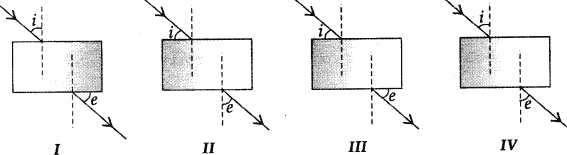(a) I
(b) II
(c) III
(d) IV

### Direction (Q 52 to Q 56): The questions given below consist of an assertion and the reason. Use the following key to choose the appropriate answer.

(a) Both the Assertion and the Reason are correct and the Reason is the correct explanation of the Assertion.
(b) The Assertion and the Reason are correct but the Reason is not the correct explanation of the Assertion.
(c) Assertion is true but the Reason is false.
(d) The statement of the Assertion is false but the Reason is true.

#### 52. Assertion: Incident light is reflected in only one direction from a smooth surface.

Reason: Since the angle of incidence and the angle of reflection are same, a beam of parallel rays of light falling on a smooth surface is reflected as a beam of parallel light rays in one direction only.

Explanation: (a) Both the Assertion and the Reason are correct and the Reason is the correct explanation of the Assertion.

#### 53. Assertion: The word AMBULANCE on the hospital vans is written in the form of its mirror as

Reason: The image formed in a plane mirror is same size of the object.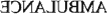Explanation: (b) The Assertion and the Reason are correct but the Reason is not the correct explanation of the Assertion.

#### 54. Assertion: Cannot see the distant object clearly.

Reason: The far point of an eye suffering j, from myopia is less than infinity.

Explanation: (b) The Assertion and the Reason are correct but the Reason is not the correct explanation of the Assertion.

#### 55. Assertion: Pupil is black in colour.

Reason: Pupil is black in colour as no light is reflected in it.

Explanation: (a) Both the Assertion and the Reason are correct and the Reason is the correct explanation of the Assertion.

#### 56. Assertion: The rainbow is a man made spectrum of sunlight in the sky.

Reason: The rainbow is formed in the sky when the sun is shining and it is raining at the same time.

Explanation: (a) The statement of the Assertion is false but the Reason is true.

#### 57. ______ mirror is used as a security mirror in shops and on roads at sharp bends and concealed entrances.

Explanation: Convex

#### 58. The refractive index of a transparent medium is the ratio of the speed of light in _____ to that in the _____ .

Explanation: vacuum, medium

#### 59. If the magnification has a minus sign, then the image is _____ and ______ .

Explanation: real, inverted

#### 60. The focal length of a lens is the distance between ______ and ______ of the lens.

Explanation: optical centre, principal focus

## Light Reflection and Refraction MCQs

#### 61. The focal length of a concave lens is considered to be ______ .

Explanation: negative

#### 62. In order to calculate the power of a lens, we need its focal length in _______ .

Explanation: metres

#### 63. Angle of refraction cannot exceed 90°. [True / False]

Explanation: True

#### 64. When incident angle i satisfies n = $$\frac{1}{\sin i}$$, the refracted light will pass along the surface. [True / False]

Explanation: True

#### 65. A person standing in front of a mirror finds his image larger than himself. This shows that mirror is convex in nature. [True / False]

Explanation: False
Convex mirror always forms a diminished image so it is false statement.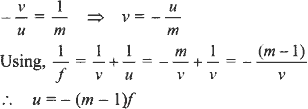i.e., the object distance from the mirror is numerically equal to (m – 1)f.

#### 66. Lateral displacement increases with the thickness of the given slab. [True / False]

Explanation: True

#### 67. Lateral displacement is directly proportional to thickness of the glass slab. [True / False]

Explanation: True

#### 68. Nature of image formed by mirror gives an ideal about nature of mirror. [True / False]

Explanation: True

#### 69. A convex lens (ng = 3/2) when placed in water (nw = 4/3) has increased focal length [True / False]

Explanation: True

#### 70. A convex and a concave lens of equal focal length behaves as a regular glass slab receiving light normally. [True / False]

Explanation: False

### Class 10 Science Light Reflection and Refraction MCQs with Answers & Explanation:

#### 71. Concave lens and convex mirror diverge the rays which fall parallel to the principal axis. [True / False]

Explanation: True

#### 72. The power of a concave lens is positive. [True / False]

Explanation: False

### Direction: Match Column I with Column II.

#### 73.

 Column I Column II (i) Ray through centre of curvature (A) Reflected parallel to principal axis (ii) Ray through focus (B) Converge at focus (iii) Rays from infinite distance (C) Emerge through focus (iv) Refracted rays to infinity (D) Retracing in mirrors

Explanation:
(i) → (D), (ii) → (A), (iii) → (B), (iv) → (C)
(i) → (D): For this ray Zi = Zr = 0
(ii) → (A): The ray of light passing through the focus will emerge parallel to the principal axis after reflection.
(iii) → (B): When object is at infinity, the image is formed at the focus of a concave mirror.
(iv) → (C): A ray from the object parallel to principal axis will pars through the principal . focus after refraction in a convex lens.

#### 74. What is light?

Explanation: Light is a form of energy. It brings the sensation of sight. It is a form of electromagnetic radiation. It also provides us means of communication (fibre-optics).

#### 75. Write any one observation from everyday life which show us that light travels in a straight line.

Explanation: A small source of light costs a sharp shadow of an opaque object tells us that light travels in a straight line.

#### 76. What is a ray?

Explanation: Ray is a line defining the path of light.

#### 77. What is beam?

Explanation: A bundle of rays originating from the same source of light in a particular direction is called beam of light.

#### 78. Explain why a ray of light passing through the centre of curvature of a concave mirror, gets reflected along the same path.

Explanation: The ray passing through the centre of curvature incident to the mirror along its normal, so ∠i = ∠r = 0. Therefore, the ray retraces its path.

#### 79. Is light a ray or a wave?

Explanation: It is considered in both the forms.

#### 80. What are the characters associated with light as a wave?

Explanation: Frequency and wavelength.

## Light Reflection and Refraction MCQs

#### 81. What is Spherical mirror?

Explanation: A reflecting surface which is of the form of a sphere (hollow) in which inner or outer surface is reflecting.

#### 82. What is the relation connecting focal length and radius of a spherical mirror?

Explanation: F = $$\frac{R}{2}$$

#### 83. Can any spherical surface act as a reflector?

Explanation: Yes, polished surface can be better reflectors.

#### 84. Redraw the diagram given below in your answer book and show the direction of the light ray after reflection from the mirror.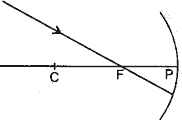Explanation: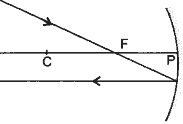#### 85. For what position of object, a concave mirror forms a real image equal to size of object?

Explanation: When object is placed at the centre of curvature (C) same size real image is formed by concave mirror.

#### 86. A concave mirror forms a sharp image of a distant tree. What name is given to the distance between the concave mirror and screen on which sharp image is formed?

Explanation: Focal length

#### 87. In what condition, the image formed by a concave mirror is virtual?

Explanation: When the object is placed between the focus and the pole of a concave mirror, a virtual image is obtained.

#### 88. Specified the size of image formed by a concave mirror when m > 1.

Explanation: The image is enlarged.

#### 89. Name the mirror that can be used to check theft in shops.

Explanation: Convex mirror.

#### 90. What is the position of the object placed on the side of reflecting surface of a concave mirror of focal length 15 cm if the image is formed at the distance of 30 cm from the mirror?

Explanation: 30 cm

### Class 10 Science Light Reflection and Refraction MCQs with Answers & Explanation:

#### 91. Which mirror, concave or convex always converges the light rays?

Explanation: Concave mirror.

#### 92. For what position of the object does a concave mirror forms a real image which is highly enlarged?

Explanation: At F.

#### 93. When object is placed at centre of centre of curvature of concave mirror, where is the image formed?

Explanation: At C.

#### 94. What focal length can be assigned to a plane mirror?

Explanation: Infinity